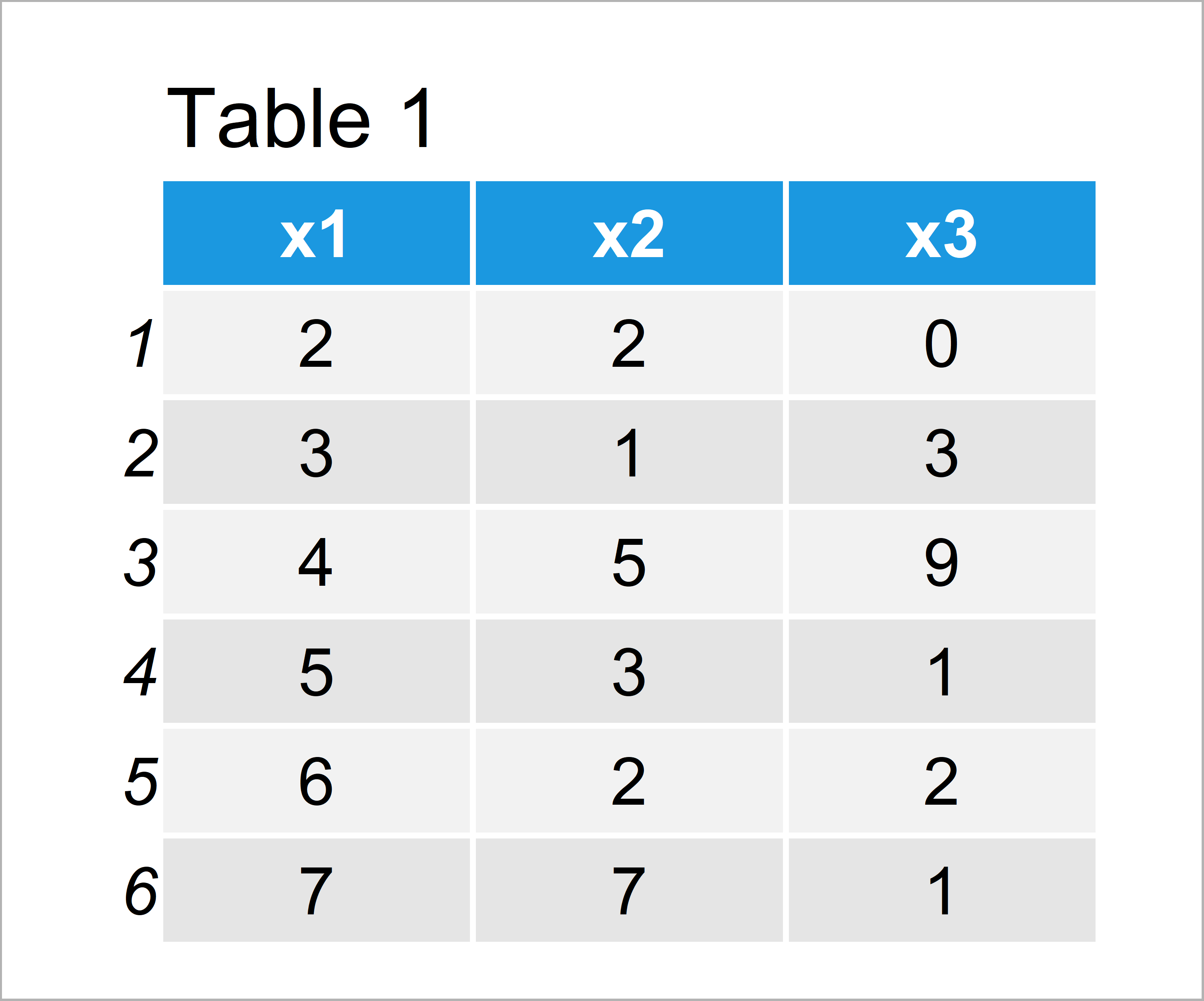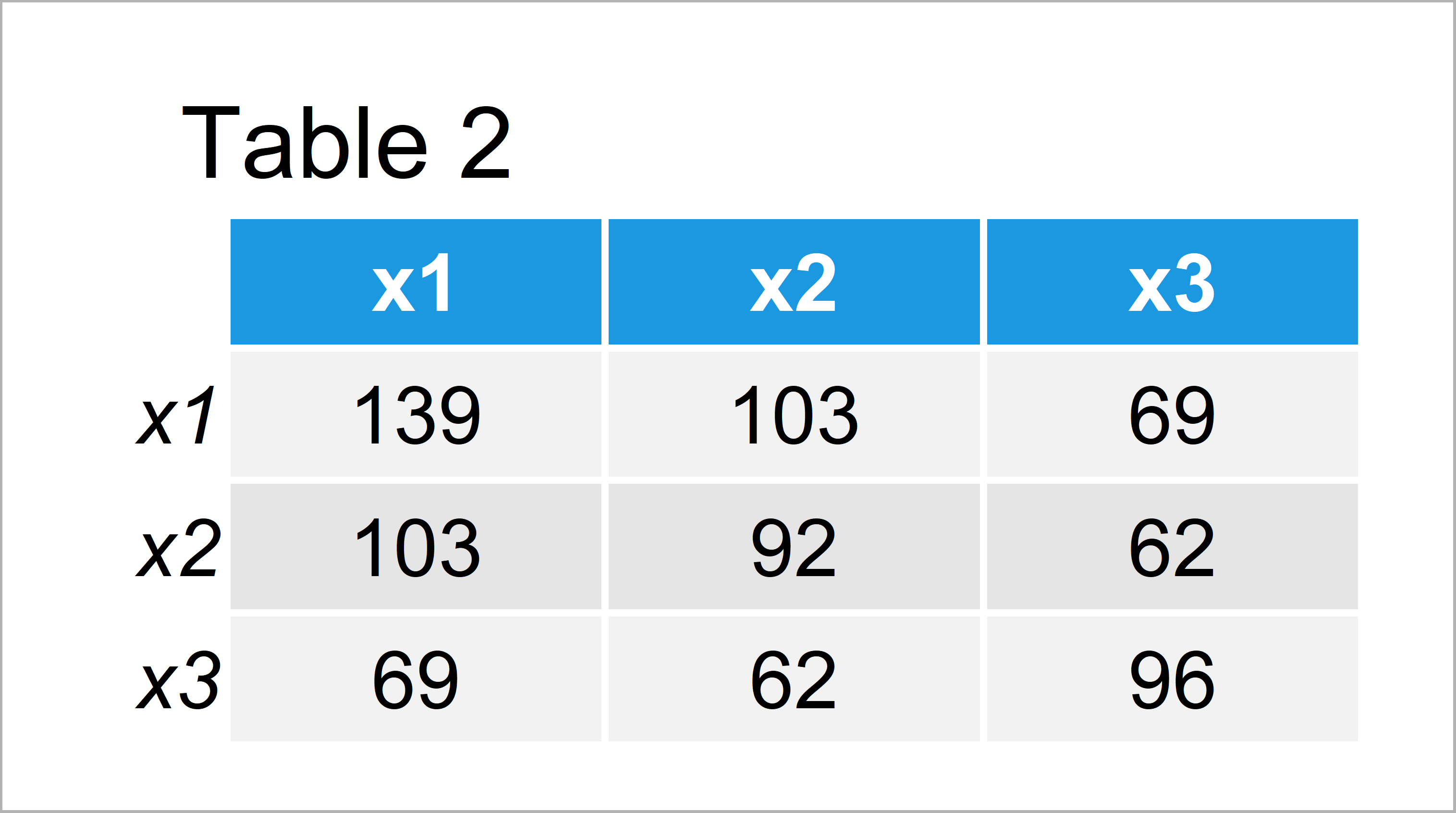# R Error – Multiplication requires numeric/complex matrix/vector arguments

In this R tutorial you’ll learn how to handle the error message “requires numeric/complex matrix/vector arguments”.

The post is structured as follows:

Let’s dive right in:

## Creation of Example Data

```data <- data.frame(x1 = 2:7,                 # Create example data
x2 = c(2, 1, 5, 3, 2, 7),
x3 = c(0, 3, 9, 1, 2, 1))
data                                         # Print example data```Table 1 shows the structure of our example data: It contains six rows and three columns.

## Example 1: Reproduce the Error – requires numeric/complex matrix/vector arguments

In this example, I’ll explain how to replicate the error message “requires numeric/complex matrix/vector arguments” in R.

Let’s assume that we want to perform a matrix multiplication based on our data. Then, we might try to use the following R syntax:

```data_multi <- t(data) %*% data               # Try to use data frame
# Error in t(data) %*% data :
#   requires numeric/complex matrix/vector arguments```

As you can see, the RStudio console returns the error message “requires numeric/complex matrix/vector arguments”. The reason for this is that our input data has the data.frame class instead of the matrix class. Since the %*% operator cannot handle data frames, it returns an error message.

So how can we solve this problem? That’s what I’ll show next!

## Example 2: Fix the Error – requires numeric/complex matrix/vector arguments

This example illustrates how to avoid the matrix multiplication error “requires numeric/complex matrix/vector arguments”.

For this, we have to convert our data frame object to the matrix type using the as.matrix function:

```data_multi <- t(data) %*% as.matrix(data)    # Convert data frame to matrix
data_multi                                   # Print result```Table 2 shows the output of the previous syntax: The result of our matrix multiplication.

## Video, Further Resources & Summary

Would you like to know more about errors and warning messages? Then you might have a look at the following video of my YouTube channel. In the video, I illustrate the contents of this tutorial.

Besides the video, you could have a look at the related tutorials on my website. Some articles are listed here.

On this page, I have shown how to deal with the error “requires numeric/complex matrix/vector arguments” in the R programming language. Don’t hesitate to let me know in the comments, in case you have additional questions and/or comments.

Subscribe to the Statistics Globe Newsletter

•Michael Urroz
June 8, 2022 8:09 pm

I am working with the harvad database on german credits for a credit scoring model. I wanted to apply the “nuralnet” function but it gives me the following error: error in neurons[[i]] %*% weights[[i]] : requires numeric/complex matrix/vector arguments.
Taking the advice from your article to the base dedicated to training, I applied:
data_train<- t(train) %*% as.matrix(train) .
but it still gives me the same error.

•June 9, 2022 6:19 am